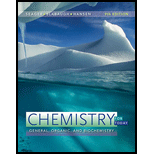# Calculate the molar concentration of H 3 O + in water solutions with the following OH − molar concentrations: a. 6.9 × 10 − 5 b. 0.074 c. 4.9 d. 1.7 × 10 − 3 e. 9.2 × 10 − 9### Chemistry for Today: General, Orga...

9th Edition
Spencer L. Seager + 2 others
Publisher: Cengage Learning
ISBN: 9781305960060

#### Solutions

Chapter
Section### Chemistry for Today: General, Orga...

9th Edition
Spencer L. Seager + 2 others
Publisher: Cengage Learning
ISBN: 9781305960060
Chapter 9, Problem 9.30E
Textbook Problem
1 views

## Calculate the molar concentration of H 3 O + in water solutions with the following OH − molar concentrations:a. 6.9 × 10 − 5 b. 0.074 c. 4.9 d. 1.7 × 10 − 3 e. 9.2 × 10 − 9

Interpretation Introduction

(a)

Interpretation:

The molar concentration of H3O+ in water solutions with the given OH molar concentrations is to be calculated.

Concept introduction:

The water undergoes self-ionization which can be represented by the reaction,

H2O(l)+H2O(l)H3O+(aq)+OH(aq)

The ionization constant of water is represented as,

K=[H3O+][OH][H2O][H2O]

The concentration of water remains constant and the self-ionization constant of water becomes,

Kw=[H3O+][OH]Kw=(1.0×107mol/L)(1.0×107mol/L)Kw=1.0×1014(mol/L)2

### Explanation of Solution

The ionic product of water Kw is,

Kw=[H3O+][OH]

The value of Kw is 1.0×1014(mol/L)2.

The given OH molar concentration is 6.9×105mol/L. Substitute this value in the formula for ionic product.

1.0×1014(mol/L)2=[H3O+]6

Interpretation Introduction

(b)

Interpretation:

The molar concentration of H3O+ in water solutions with the given OH molar concentrations is to be calculated.

Concept introduction:

The water undergoes self-ionization which can be represented by the reaction,

H2O(l)+H2O(l)H3O+(aq)+OH(aq)

The ionization constant of water is represented as,

K=[H3O+][OH][H2O][H2O]

The concentration of water remains constant and the self-ionization constant of water becomes,

Kw=[H3O+][OH]Kw=(1.0×107mol/L)(1.0×107mol/L)Kw=1.0×1014(mol/L)2

Interpretation Introduction

(c)

Interpretation:

The molar concentration of H3O+ in water solutions with the given OH molar concentrations is to be calculated.

Concept introduction:

The water undergoes self-ionization which can be represented by the reaction,

H2O(l)+H2O(l)H3O+(aq)+OH(aq)

The ionization constant of water is represented as,

K=[H3O+][OH][H2O][H2O]

The concentration of water remains constant and the self-ionization constant of water becomes,

Kw=[H3O+][OH]Kw=(1.0×107mol/L)(1.0×107mol/L)Kw=1.0×1014(mol/L)2

Interpretation Introduction

(d)

Interpretation:

The molar concentration of H3O+ in water solutions with the given OH molar concentrations is to be calculated.

Concept introduction:

The water undergoes self-ionization which can be represented by the reaction,

H2O(l)+H2O(l)H3O+(aq)+OH(aq)

The ionization constant of water is represented as,

K=[H3O+][OH][H2O][H2O]

The concentration of water remains constant and the self-ionization constant of water becomes,

Kw=[H3O+][OH]Kw=(1.0×107mol/L)(1.0×107mol/L)Kw=1.0×1014(mol/L)2

Interpretation Introduction

(e)

Interpretation:

The molar concentration of H3O+ in water solutions with the given OH molar concentrations is to be calculated.

Concept introduction:

The water undergoes self-ionization which can be represented by the reaction,

H2O(l)+H2O(l)H3O+(aq)+OH(aq)

The ionization constant of water is represented as,

K=[H3O+][OH][H2O][H2O]

The concentration of water remains constant and the self-ionization constant of water becomes,

Kw=[H3O+][OH]Kw=(1.0×107mol/L)(1.0×107mol/L)Kw=1.0×1014(mol/L)2

### Still sussing out bartleby?

Check out a sample textbook solution.

See a sample solution

#### The Solution to Your Study Problems

Bartleby provides explanations to thousands of textbook problems written by our experts, many with advanced degrees!

Get Started

Find more solutions based on key concepts
Does the cell cycle refer to mitosis as well as meiosis?

Human Heredity: Principles and Issues (MindTap Course List)

For healthy adults, the DRI recommended intake for protein has been set at 0.3 grams per kilogram of body weigh...

Nutrition: Concepts and Controversies - Standalone book (MindTap Course List)

By what factor is the capacitance of a metal sphere multiplied if its volume is tripled? (a) 3 (b) 3 (c) 1 (d) ...

Physics for Scientists and Engineers, Technology Update (No access codes included)